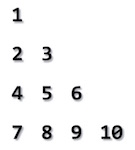Random Coding

Infinite recursion

Write a function add such that you can call it like this:
``````console.log(add(1)); // logs 1

Multiplicative anagram

Write a program to find the smallest number such that if you multiply it by the numbers 1-x, each result will have all the same digits. For example, a number that works when x is 2, is 285714.
``````1 * 285714 === 285714
2 * 285714 === 571428``````
Both numbers have the digits 2, 8, 5, 7, 1, 4

Random order

Generate all random numbers between a, b without repetition e.g. randm(1, 7) -> 1,2,3,4,5,6,7

Random from to  ↻

Floyd triangle

Write a function that print's a triangle of natural numbers based on the number of row requested. For example, if 4 rows are requested, it looks like:How many rows: